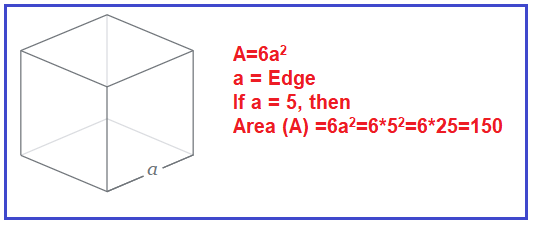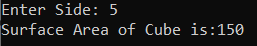# Surface Area of Cube in C#

## Surface Area of Cube Program in C# with Examples

In this article, I am going to discuss how to calculate the Surface Area of Cube in C# with Examples. Please read our previous article, where we discussed the Area of Circle Program in C#. Here, in this article, first, we will discuss what is Cube, then we will discuss how to calculate the area of a Cube and finally, we will see how to implement the area of cube program in C#.

##### What is a Cube?

A cube is a 3D (Three Dimensional) shape. It has six faces, 8 vertices, and 12 edges and all the six faces of a cube are squares.
Surface Area of Cube (SA)= 6* side *side = 6a2

Where a is the side of the cube
SA is the surface area of the cube

###### Example:

Input: Edge of a cube = 5

Surface Area (SA)= 6a2 = 6 × 52 = 6 × 25  = 150##### Logic to Calculate the Surface Area of Cube in C#

To find the surface area of the cube, we need to follow the below steps

1. First, we will define variable, Side, Area.
2. Then we will calculate the surface area of the cube by formula. 6* Side * Side
3. And the result will be store in Area.
##### Example: Surface Area of Cube Program in C#

The following C# Program will calculate the surface area of the cube.

```using System;
class AreaOfCube
{
static void Main()
{
Console.Write("Enter Side: ");